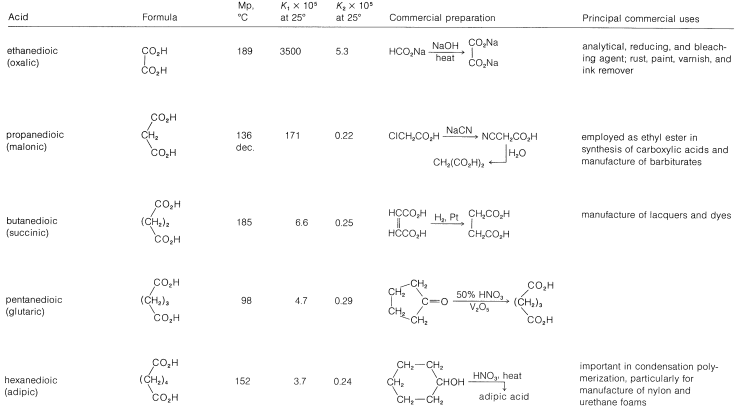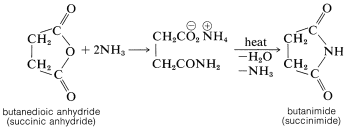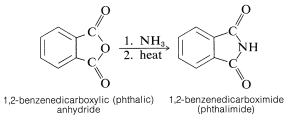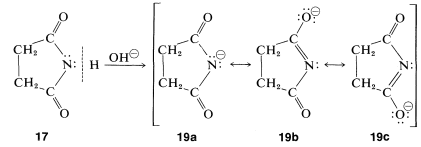# 18.11: Dicarboxylic Acids

$$\newcommand{\vecs}{\overset { \rightharpoonup} {\mathbf{#1}} }$$ $$\newcommand{\vecd}{\overset{-\!-\!\rightharpoonup}{\vphantom{a}\smash {#1}}}$$$$\newcommand{\id}{\mathrm{id}}$$ $$\newcommand{\Span}{\mathrm{span}}$$ $$\newcommand{\kernel}{\mathrm{null}\,}$$ $$\newcommand{\range}{\mathrm{range}\,}$$ $$\newcommand{\RealPart}{\mathrm{Re}}$$ $$\newcommand{\ImaginaryPart}{\mathrm{Im}}$$ $$\newcommand{\Argument}{\mathrm{Arg}}$$ $$\newcommand{\norm}{\| #1 \|}$$ $$\newcommand{\inner}{\langle #1, #2 \rangle}$$ $$\newcommand{\Span}{\mathrm{span}}$$ $$\newcommand{\id}{\mathrm{id}}$$ $$\newcommand{\Span}{\mathrm{span}}$$ $$\newcommand{\kernel}{\mathrm{null}\,}$$ $$\newcommand{\range}{\mathrm{range}\,}$$ $$\newcommand{\RealPart}{\mathrm{Re}}$$ $$\newcommand{\ImaginaryPart}{\mathrm{Im}}$$ $$\newcommand{\Argument}{\mathrm{Arg}}$$ $$\newcommand{\norm}{\| #1 \|}$$ $$\newcommand{\inner}{\langle #1, #2 \rangle}$$ $$\newcommand{\Span}{\mathrm{span}}$$

Acids in which there are two carboxyl groups separated by a chain of more than five carbon atoms $$\left( n > 5 \right)$$ for the most part have unexceptional properties, and the carboxyl groups behave more or less independently of one another.However, when the carboxyl groups are closer together the possibilities for interaction increase; we shall be interested primarily in such acids. A number of important dicarboxylic acids are listed in Table 18-4 together with their physical properties, methods of manufacture, and commercial uses.

Table 18-4: Dicarboxylic Acids## Acidic Properties of Dicarboxylic Acids

The inductive effect of one carboxyl group is expected to enhance the acidity of the other. In Table 18-4 we see that the acid strength of the dicarboxylic acids, as measured by the first acid-dissociation constant, $$K_1$$, is higher than that of ethanoic acid $$\left( K_\text{a} = 1.5 \times 10^{-5} \right)$$ and decreases with increasing number of bonds between the two carboxyl groups. The second acid-dissociation constant, $$K_2$$, is smaller than $$K_\text{a}$$ for ethanoic acid (with the exception of oxalic acid) because it is more difficult to remove a proton under the electrostatic attraction of the nearby carboxylate anion (see Section 18-2C).

## Thermal Behavior of Dicarboxylic Acids

The reactions that occur when dicarboxylic acids are heated depend critically upon the chain length separating the carboxyl groups. Cyclization usually is favored if a strainless five- or six-membered ring can be formed. Thus hexanedioic and heptanedioic acids decarboxylate and cyclize to cyclopentanone and cyclohexanone, respectively:Butanedioic and pentanedioic acids take a different course. Rather than form the strained cyclic ketones, cyclopropanone and cyclobutanone, both acids form cyclic anhydrides that have five- and six-membered rings, respectively. 1,2-Benzenedicarboxylic (phthalic) and cis-1,4-butenedicarboxylic (maleic) acids behave similarly:Because of their short chains, propanedioic and ethanedioic acids simply decarboxylate when heated (Section 18-4):## Imides from Dicarboxylic Acids

The cyclic anhydride of butanedioic acid reacts with ammonia, as may be expected for a typical anhydride; but the product, when strongly heated, forms a cyclic imide (butanimide):1,2-Benzenedicarboxylic (phthalic) anhydride behaves similarly, giving 1,2-benzenedicarboximide (phthalimide):Unlike amines, imides do not have basic properties in water solution; the electron pair of nitrogen is partly delocalized over the carbonyl groups, as indicated by $$17a$$ to $$17c$$. This stabilization is lost if a proton is added to nitrogen to give the conjugate acid, $$18$$:Imides are, in fact, quite acidic and readily dissolve in alkali-metal hydroxide solutions to give salts. Like carboxylic acids and 1,3-dicarbonyl compounds, imides are acidic primarily because the stabilization of the anion is greater than that of the acid. This can be seen by comparison of the resonance structures that may be written for the imide, $$17$$, with those of the anion, $$18$$. Separation of positive and negative charge, as in Structures $$17b$$ and $$17c$$, increases the energy of such structures. There is no charge separation in the anion; thus $$19b$$ and $$19c$$ are more important with respect to their hybrid than are $$17b$$ and $$17c$$ to their hybrid. (You may wish to review the corresponding argument for the acidity of carboxylic acids, Section 18-2A.)The salts of imides are useful in synthesis, as is described in Section 23-9D.

## The Dieckmann Condensation

Esters of most dicarboxylic acids, except propanedioic esters, undergo Claisen condensation in much the same way as do esters of monocarboxylic acids (see Section 18-8B). However, when a strainless five- or six-membered ring can be formed, an intramolecular Claisen condensation, called the Dieckmann condensation, may take place which would result in the formation of a cyclic $$\beta$$-keto ester:## The Acyloin Reaction

A useful method of forming carbon-carbon bonds involves reduction of esters with sodium metal in aprotic solvents such as ether or benzene and is called the acyloin reaction:This interesting reaction is especially useful for the synthesis of medium- and large-ring compounds from dicarboxylic esters, and is effective for ring sizes that cannot be made by the Dieckmann condensation or decarboxylation (Section 18-10B). Radical anions formed by addition of sodium to the ester groups appear to be the key intermediates for carbon-carbon bond formation. Thus, for dimethyl decanedioate: